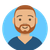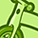New to Qlik Sense

If you’re new to Qlik Sense, start with this Discussion Board and get up-to-speed quickly.

Announcements
QLIKWORLD LIVE! MAY 16 - 19TH, EARLY BIRD DISCOUNTS! REGISTER TODAY
cancel
Showing results for
Did you mean:Creator II

how to ignore the selection of one dimension in a multiple set analysis

Dear All,

I have an set analysis calculation like below in a table,  and i want to disregard the selection of the dimension of fiscal year in this case.  and i had tried in different ways like below but it didn't work, can you help??

Sum({<[Order Type]={'Y470','Y471'},DateType={'Order'},Order_Material_Pricing_Group_Text={'Lab machines', 'machines', 'Mills'}, [Material Number]-={'NSCMACHINE', 'NSCTANK'}>} Aggr(Order_Item_Quantity,[Order No.],[Item Number]))

Trial 1:

Sum({<[Order Type]={'Y470','Y471'},DateType={'Order'},FiscalYear=,Order_Material_Pricing_Group_Text={'Lab machines', 'machines', 'Mills'}, [Material Number]-={'NSCMACHINE', 'NSCTANK'}>} Aggr(Order_Item_Quantity,[Order No.],[Item Number]))

Trial 2:

Sum({<[Order Type]={'Y470','Y471'},DateType={'Order'},Order_Material_Pricing_Group_Text={'Lab machines', 'machines', 'Mills'}, [Material Number]-={'NSCMACHINE', 'NSCTANK'}>} <Total FiscalYear=>,Aggr(Order_Item_Quantity,[Order No.],[Item Number]))

Labels (1)
• selection of dimension

1 Solution

Accepted SolutionsPartner

You need the set logic inside the Aggr() as well - something like:

Sum({<
[Order Type]={'Y470','Y471'},
DateType={'Order'},
FiscalYear=,
Order_Material_Pricing_Group_Text={'Lab machines', 'machines', 'Mills'},
[Material Number]-={'NSCMACHINE', 'NSCTANK'}
>}

Aggr(Only(
{<
[Order Type]={'Y470','Y471'},
DateType={'Order'},
FiscalYear=,
Order_Material_Pricing_Group_Text={'Lab machines', 'machines', 'Mills'},
[Material Number]-={'NSCMACHINE', 'NSCTANK'}
>}
Order_Item_Quantity)
,[Order No.],[Item Number])
)
Logic will get you from a to b. Imagination will take you everywhere. - A Einstein
11 RepliesPartner

Why do you need aggr after sum?

Expression should looks like below if you need aggr function:

Aggr(Sum({<[Order Type]={'Y470','Y471'},DateType={'Order'},FiscalYear=,Order_Material_Pricing_Group_Text={'Lab machines', 'machines', 'Mills'}, [Material Number]-={'NSCMACHINE', 'NSCTANK'}>} Order_Item_Quantity),[Order No.],[Item Number])Creator II
Author

the reason of putting aggr() after Sum is because, there are repeated lines in the original source file, so that we need to use aggr() firstly to define the unique line then sum.

however the point here is how to disregard the selection of the dimension of FiscalYear in the set analysis, and i had tried in your way like my trial 1 indicated, but it didn't work. If I selected certain fiscal year, the table results still changes as per my selection, how to keep them fixed??Partner

You have a sample app?Partner

Can you try:

Sum({<[Order Type]={'Y470','Y471'},DateType={'Order'},FiscalYear=,Order_Material_Pricing_Group_Text={'Lab machines', 'machines', 'Mills'}, [Material Number]-={'NSCMACHINE', 'NSCTANK'}>} distinct Order_Item_Quantity)Luminary Alumni

I think anything else associated with FISCAL_YEAR that may be filtered as a result also needs to be set the way you have with FISCAL_YEAR. There's likely other fields in your app that are affected.Creator II
Author

@Arthur_Fong  thanks.

unfortunately i have no sample app so far. the order_item_quantity cannot be written in this way, because it was also linked with which order number it relates, so you has to keep my original structure like that: Sum(aggr(...)),

with "Fiscalyear= " added into the set analysis it doesn't work out.Creator II
Author

@Rodj  what do you exactly mean here? can you explain in details? and further how to resolve this? thanksPartner

You need the set logic inside the Aggr() as well - something like:

Sum({<
[Order Type]={'Y470','Y471'},
DateType={'Order'},
FiscalYear=,
Order_Material_Pricing_Group_Text={'Lab machines', 'machines', 'Mills'},
[Material Number]-={'NSCMACHINE', 'NSCTANK'}
>}

Aggr(Only(
{<
[Order Type]={'Y470','Y471'},
DateType={'Order'},
FiscalYear=,
Order_Material_Pricing_Group_Text={'Lab machines', 'machines', 'Mills'},
[Material Number]-={'NSCMACHINE', 'NSCTANK'}
>}
Order_Item_Quantity)
,[Order No.],[Item Number])
)
Logic will get you from a to b. Imagination will take you everywhere. - A EinsteinContributor

It is however very simple at heart. You can add a Set to an expression which changes the selection context for that expression – effectively forcing selections that only apply to that one expression. So sum(Value) becomes sum({<Type={‘Relevant’}>}Value), if you only want to add up the values which are flagged as being Relevant in the data myprepaidcenterTags
Community Browser